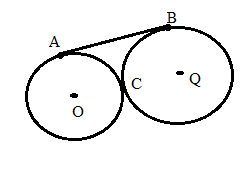Chapter 6.3, Problem 37E### Elementary Geometry for College St...

6th Edition
Daniel C. Alexander + 1 other
ISBN: 9781285195698

#### Solutions

Chapter
Section### Elementary Geometry for College St...

6th Edition
Daniel C. Alexander + 1 other
ISBN: 9781285195698
Textbook Problem
1 views

# Given: A B ¯ is an external tangent to ⊙ O and ⊙ Q at points A and B; radii lengths for ⊙ O and ⊙ Q are 4 and 9.Find: AB(HINT: The line of centers O Q ¯ contains point C, the point at which ⊙ O and ⊙ Q are tangent.)

To determine

To find:

The find the value of AB.

Explanation

Given that, AB¯ is an external tangent to O and Q at points A and B; radii lengths for O and Q are 4 and 9.

The line of centers OQ¯ contains the point C in which the circles are internally tangent. Then draw the tangent and join O, Q with P.

The diagrammatic representation is given below,

From the above graph PA¯,PC¯ tangents from the exterior point P to circle O. That is, PO¯ bisects APC therefore, PA=PC.

From the above graph PB¯,PC¯ tangents from the exterior point P to circle Q. That is, PQ¯ bisects CPB therefore, PC=PB.

Then add the angles to get the following,

mOPQ=mOPC+mQPC=mAPC2+mCPB2=mAPC+mCPB2=90+90"

### Still sussing out bartleby?

Check out a sample textbook solution.

See a sample solution

#### The Solution to Your Study Problems

Bartleby provides explanations to thousands of textbook problems written by our experts, many with advanced degrees!

Get Started